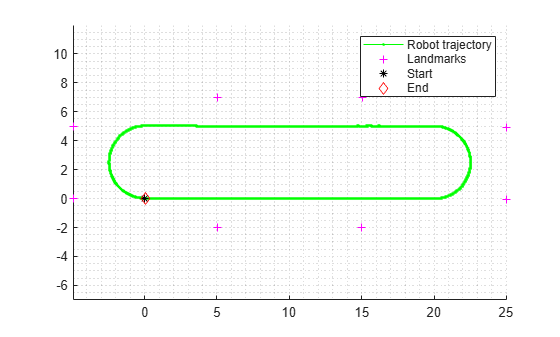# correct

Correct state and state error covariance

Since R2021b

## Syntax

``[associations,newLandmark] = correct(slamObj,measurement,measurementCovariance)``
``[associations,newLandmark] = correct(slamObj,measurement,measurementCovariance,varargin)``

## Description

````[associations,newLandmark] = correct(slamObj,measurement,measurementCovariance)` corrects the state and its associated state covariance based on the `measurement` and `measurementCovariance` at the current time step. `correct` uses the data association function specified in the DataAssociationFcn property of the `ekfSLAM` object, `slamObj`, to associate the measurement to landmarks and extract new landmarks from the measurement.The `correct` function uses these associations to correct the state and associated state covariance, then augments the state with new landmarks.```

example

````[associations,newLandmark] = correct(slamObj,measurement,measurementCovariance,varargin)` passes all additional arguments specified in `varargin` to the underlying DataAssociationFcn property of `slamObj`.The first four inputs to the `DataAssociationFcn` property are the landmark position, landmark position covariance, measurement, and measurement covariance, followed by all arguments in `varargin`.```

## Examples

collapse all

Load a race track data set that contains the initial vehicle state, initial vehicle state covariance, process noise covariance, control input, time step size, measurement, measurement covariance, and validation gate values.

```load("racetrackDataset.mat","initialState","initialStateCovariance", ... "processNoise","controllerInputs","timeStep", ... "measurements","measCovar","validationGate");```

Create an `ekfSLAM` object with initial state, initial state covariance, and process noise.

```ekfSlamObj = ekfSLAM("State",initialState, ... "StateCovariance",initialStateCovariance, ... "ProcessNoise",processNoise);```

Initialize a variable to store the pose.

```storedPose = nan(size(controllerInputs,1)+1,3); storedPose(1,:) = ekfSlamObj.State(1:3);```

Predict the state using the control input and time step size for the state transition function. Then, correct the state using the data of the observed landmarks, measurement covariance, and validation gate for the data association function.

```for count = 1:size(controllerInputs,1) % Predict the state predict(ekfSlamObj,controllerInputs(count,:),timeStep); % Get the landmarks in the environment observedLandmarks = measurements{count}; % Correct the state if ~isempty(observedLandmarks) correct(ekfSlamObj,observedLandmarks, ... measCovar,validationGate); end % Log the estimated pose storedPose(count+1,:) = ekfSlamObj.State(1:3); end```

Visualize the created map.

```fig = figure; figAx = axes(fig); axis equal grid minor hold on plot(figAx,storedPose(:,1),storedPose(:,2),"g.-") landmarks = reshape(ekfSlamObj.State(4:end),2,[])'; plot(figAx,landmarks(:,1),landmarks(:,2),"m+") plot(figAx,storedPose(1,1),storedPose(1,2),"k*") plot(figAx,storedPose(end,1),storedPose(end,2),"rd") legend("Robot trajectory","Landmarks","Start","End")```## Input Arguments

collapse all

EKF SLAM object, specified as an `ekfSLAM` object.

Measurements of the landmarks in the environment, specified as an N-by-K matrix. K is the dimension of the measurement. N is the number of measurements.

Data Types: `single` | `double`

Covariance of the measurements, specified as a K-element vector or N*K-by-N*K matrix. K is the dimension of the measurement. N is the number of measurements. When specified as a vector, the same covariance value is used for all measurements.

Data Types: `single` | `double`

Variable-length input argument list, specified as a comma-separated list. This input is passed directly into the DataAssociationFcn property of `slamObj`. When you call:

`correct(slamObj,measurement,measurementCovariance,arg1,arg2)`

MATLAB® essentially calls the `dataAssociationFcn` as:

```dataAssociationFcn(knownLandmarks,knownLandmarksCovariance, ... measurement,measurementCovariance,arg1,arg2)```

## Output Arguments

collapse all

List of associations of landmarks to measurements, returned as a P-by-2 matrix. P is the number of associations. The first column of the matrix contains the indices of the associated landmarks, and the second column contains the associated measurement indices.

List of indices of the measurements that qualify as new landmarks, returned as a Q-element vector. Q is the number of measurements that qualify as new landmarks.

## Version History

Introduced in R2021b